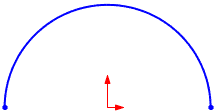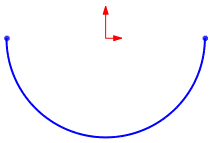Sketch Settings MenuSketch ComplexityWorking in a SketchInferencingSketch ModesAutotransitioningSelecting ContoursExiting SketchesBelt/Chain PropertyManagerCenterlinesSketching Infinite LinesLine Properties PropertyManager - 2DInsert Line PropertyManager - 2DUsing RapidSketchLink to PropertyGlossary

# Equation Driven Curves

When you create equation driven curves, the values you use must be in radians.

You cannot use global variables directly for equation driven curves. However, you can create a global variable and associate it with a dimension, then use the dimension in the equation for the curve.

• To control the curve rotation, you can drag the curve, add relations, and so on. The curve acts as any other rigid curve or block.
• To scale the curve, you need to account for scaling in the equation. For instance, in the example below, you can multiply X and Y by 10.
• To set start and end coordinates, for parametric curves, the start and end points are initially the evaluation of X and Y at T1 and T2. You can transform the curve by adding relations or dimensioning the start and end points to other sketches or model geometry.
Example of explicit equation:
 yx = sqrt( 4 - x ^ 2) x1 = -2 x2 = 2Example of parametric equation:
 xt = 50*cos(t) yt = 50*sin(t) t1 = 0 t2 = piExample of 3D equation driven curve:
 xt = 2*sin(t) yt = 2*cos(t) zt = t t1 = 0 t2 = 30Provide feedback on this topic

* Required

 *Email: Subject: Feedback on Help Topics Page: Equation Driven Curves *Comment: * I acknowledge I have read and I hereby accept the privacy policy under which my Personal Data will be used by Dassault Systèmes

Print Topic

Select the scope of content to print:

x

We have detected you are using a browser version older than Internet Explorer 7. For optimized display, we suggest upgrading your browser to Internet Explorer 7 or newer.

Never show this message again
x

Web Help Content Version: SOLIDWORKS 2013 SP05

To disable Web help from within SOLIDWORKS and use local help instead, click Help > Use SOLIDWORKS Web Help.

To report problems encountered with the Web help interface and search, contact your local support representative. To provide feedback on individual help topics, use the “Feedback on this topic” link on the individual topic page.